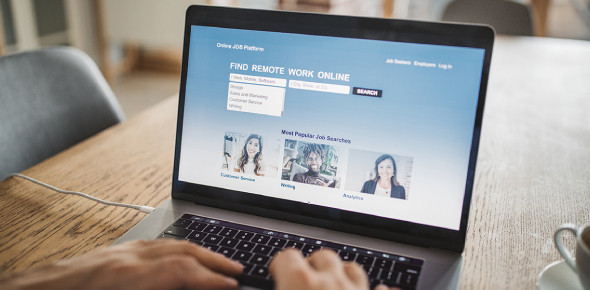# Test Your Computer Basics! Quiz

13 Questions | Attempts: 931SettingsWould you like to test your computer basics? This quiz can be of service. You communicate on the computer by utilizing the keyboard. It allows you to type letters, numbers, and words into the computer. You can also use the mouse or a trackpad on laptops. Learning how to use these items is crucial when learning to use the computer. See how much you know about your computer basics with this quiz.

• 1.
What are these examples of? -Printer -Mouse -Moniter -Keyboard -Scanner
• 2.
1,000 characters are equal to what?
• A.

1,000 bytes

• B.

1 kilobyte

• C.

1 gigabyte

• D.

1 megabyte

• 3.
What memory is Non-Volatile
• 4.
What is used for encoding the alphabet?
• A.

Bits

• B.

Barts

• C.

Bytes

• D.

Bakes

• 5.
What type is this included in? -Wordprocessing -Spreadsheets -Games -DPT
• A.

Hardware

• B.

Software

• 6.
1,000 megabytes =
• A.

1,000 gigibytes

• B.

1 gigibyte

• C.

1,000 kilobytes

• D.

1 gigabyte

• 7.
1,000 kilobytes =
• A.

1 byte

• B.

1,000 gigabytes

• C.

1 megabytes

• D.

1,000 megabytes

• 8.
What does RAM stand for?
• 9.
How many bits to make a byte?
• A.

8 bits

• B.

14 bits

• C.

5 bits

• D.

7 bits

• E.

10 bits

• 10.
Give me a characteristic of Hardware.
• 11.
What is the Binary Code?
• A.

2 and 3

• B.

4 and 5

• C.

77 and 99

• D.

6 and 7

• E.

0 and 1

• 12.
The CPU is also known as the what of the computer?
• 13.
What is the speed of the CPU?
• A.

Gerghertz

• B.

Megahertz

• C.

Bibahertz

• D.

Kilohertz

## Related TopicsBack to top
×

Wait!
Here's an interesting quiz for you.